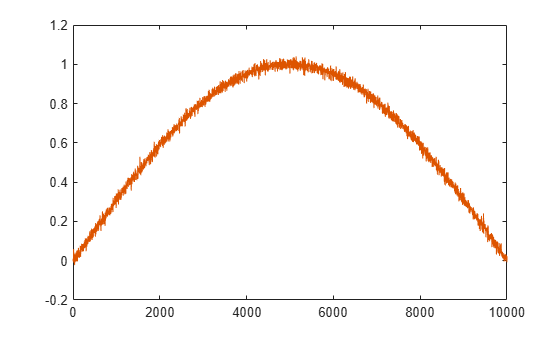# Apply Lowpass Filter to Input Signal

Assuming a sample rate of 20 kHz, create a fourth-order Butterworth filter with a 3-dB frequency of 2.5 kHz. Filter coefficients for `butter` must be constants for code generation.

`type ButterFilt`
```function output_data=ButterFilt(input_data) %#codegen [b,a]=butter(4,0.25); output_data=filter(b,a,input_data); end ```

Use the Butterworth filter to lowpass-filter a noisy sine wave.

```t = transpose(linspace(0,pi,10000)); x = sin(t) + 0.03*randn(numel(t),1);```

Filter the noisy sine wave using the Butterworth filter. Plot the filtered signal.

```fx = ButterFilt(x); plot(fx)```Run the `codegen` command to obtain the C source code `ButterFilt.c` and MEX file:

`codegen ButterFilt -args {zeros(10000,1)} -o ButterFilt_mex -report`
```Code generation successful: To view the report, open('codegen/mex/ButterFilt/html/report.mldatx') ```

The C source code includes the five numerator and denominator coefficients of the fourth-order Butterworth filter as static constants. Apply the filter using the MEX-file. Plot the filtered signal.

```output_data = ButterFilt_mex(x); hold on plot(output_data) hold off```